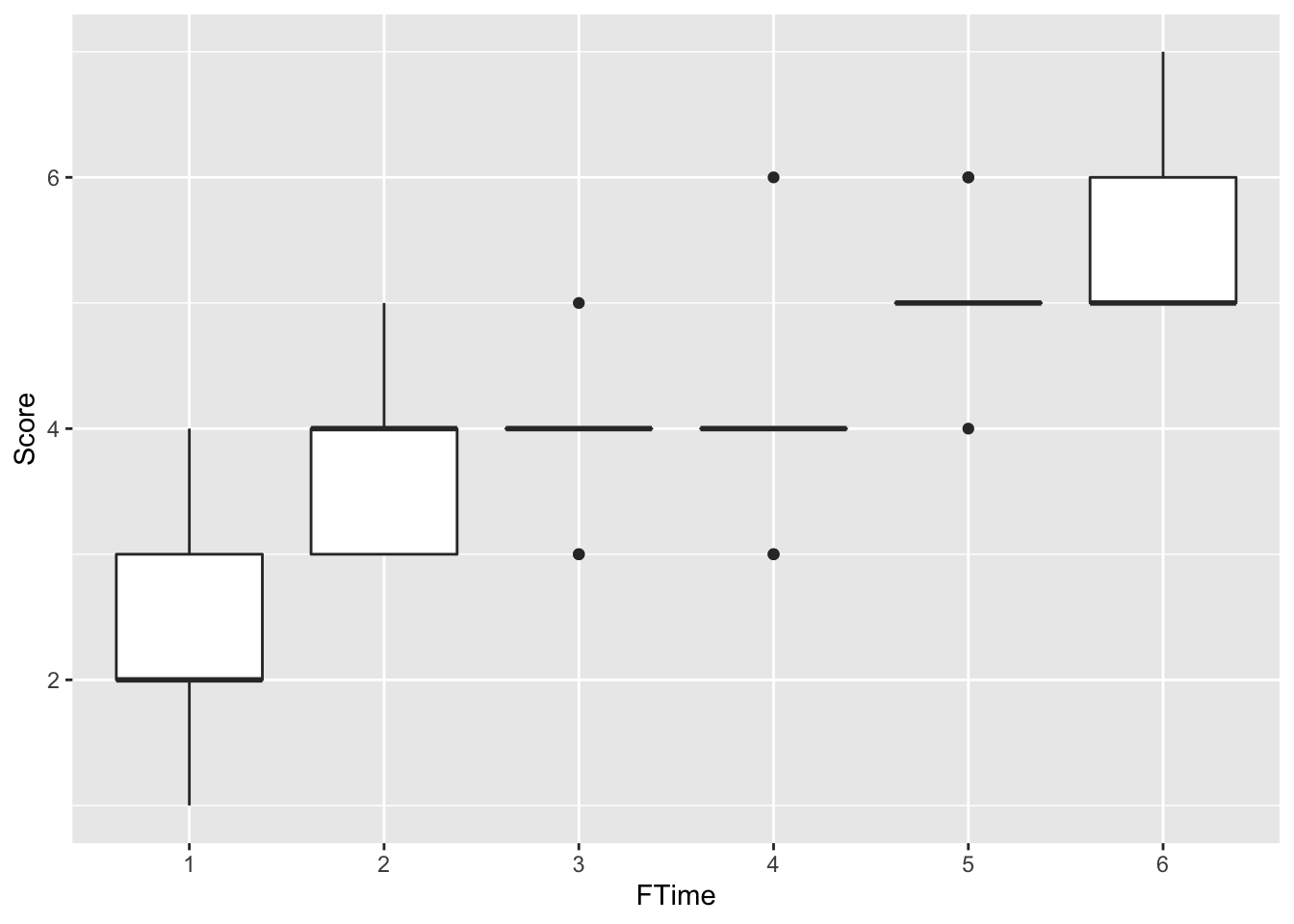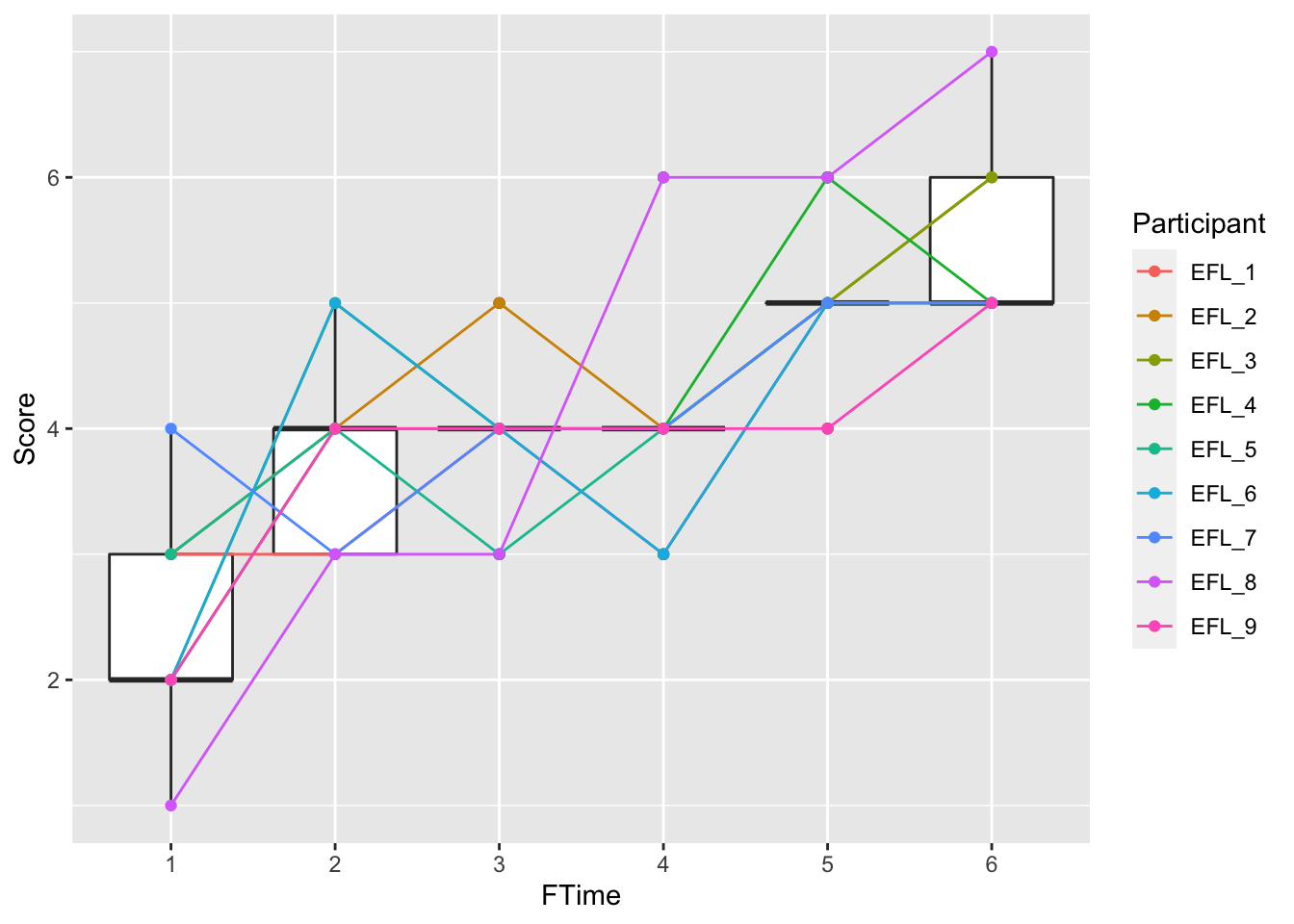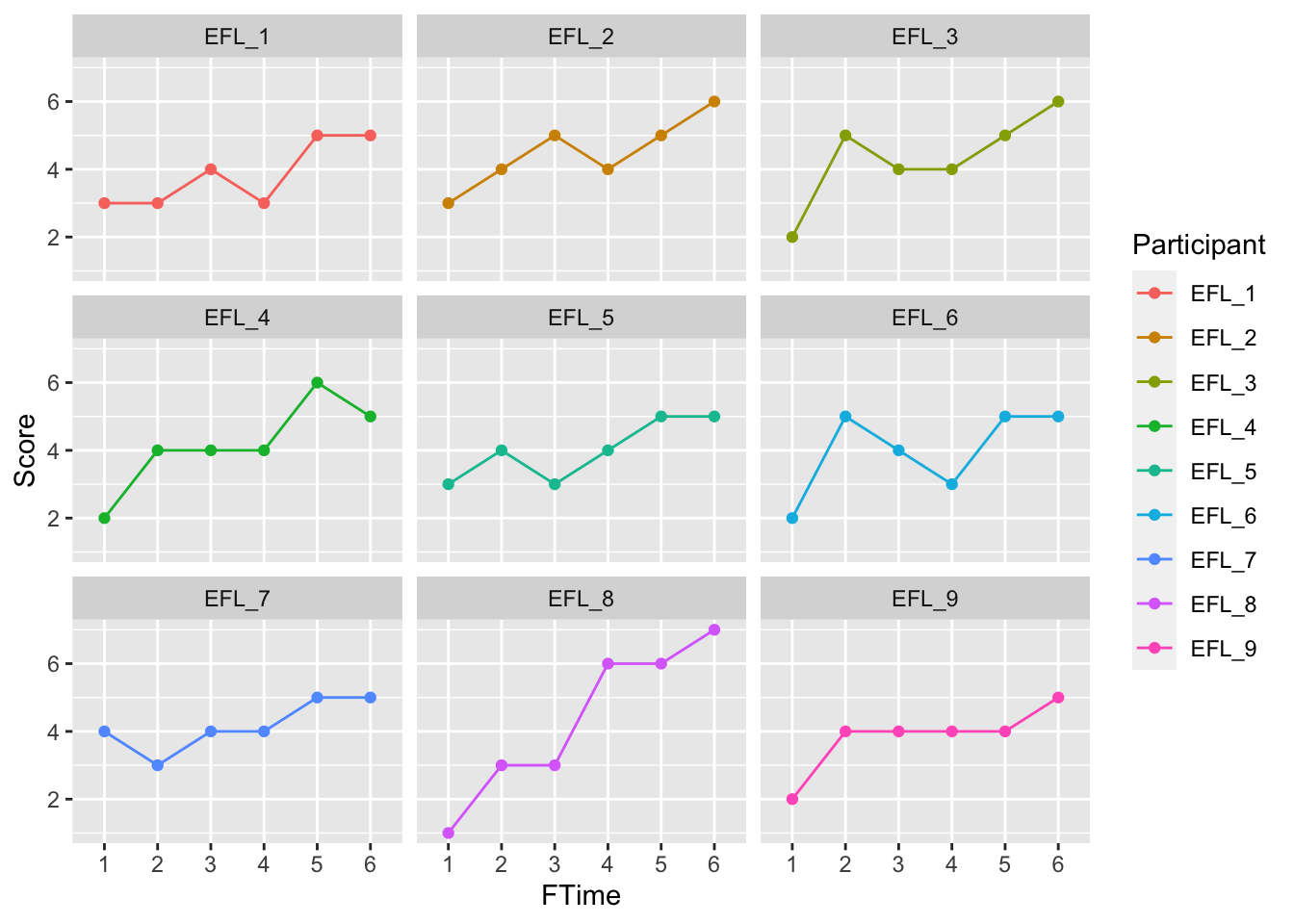## Repeated Measures ANOVA

This tutorial will cover the repeated measures analysis of variance (ANOVA) test, which has traditionally been used as the multivariate (or multi-group) alternative to the paired samples t-test. Note that newer, arguably better methods are currently being used as well, namely linear mixed-effects models (these will be covered in the next tutorial). Accordingly, this tutorial will be rather brief.

### Description of sample data

This data comprises L2 English essays written over a two year period by nine middle-school aged Dutch children studying at an English/Dutch bilingual school in the Netherlands. Essays were collected three times a year (roughly every four months) over two academic years. Included in the dataset are holistic scores for each essay (“Score”) and mean length of T-unit (MLT) values. In this tutorial, we will explore the relationship between holistic scores and time spent studying English, with the alternative hypothesis that holistic essay scores will increase as a function of time. For further reference, see Kyle (2016).

``````mydata <- read.csv("data/RM_sample.csv", header = TRUE)
#First, we create a new variable that is the categorical version of Time
mydata\$FTime <- factor(mydata\$Time)
summary(mydata)``````
``````##   Participant      Time         Score           MLT         FTime
##  EFL_1  : 6   Min.   :1.0   Min.   :1.00   Min.   : 6.895   1:9
##  EFL_2  : 6   1st Qu.:2.0   1st Qu.:3.00   1st Qu.: 9.438   2:9
##  EFL_3  : 6   Median :3.5   Median :4.00   Median :10.976   3:9
##  EFL_4  : 6   Mean   :3.5   Mean   :4.13   Mean   :11.517   4:9
##  EFL_5  : 6   3rd Qu.:5.0   3rd Qu.:5.00   3rd Qu.:12.906   5:9
##  EFL_6  : 6   Max.   :6.0   Max.   :7.00   Max.   :18.889   6:9
##  (Other):18``````

### Visualizing the data

First, we can look at the means at each time point.

``````library(ggplot2)
ggplot(data = mydata, aes(x = FTime, y = Score, group = Time)) +
geom_boxplot()``````Then, we can get a clearer view by looking at individual trajectories.

``````library(ggplot2)
ggplot(data = mydata, aes(x = FTime, y = Score, group = Participant)) +
geom_boxplot(aes(group=Time)) +
geom_line(aes(color=Participant)) +
geom_point(aes(color=Participant))``````Sometimes, we get a clearer view of individual trajectories when we use facet wrap:

``````ggplot(data = mydata, aes(x = FTime, y = Score, group = Participant)) +
geom_line(aes(color=Participant)) +
geom_point(aes(color=Participant)) +
facet_wrap(~Participant)``````### Conducting repeated measures ANOVA

Below, we conduct the repeated measures ANOVA. Note that this is almost the same as an independent one-way ANOVA, but there is one addition. We account for that fact that each participant wrote multiple essays in our sample by adding an “Error” term (in this case, “Participant”). As we can see, there are significant differences between at least two of our groups (p = 7.2e-09).

``````rm_anova <- aov(Score~FTime + Error(Participant), data=mydata)
summary(rm_anova)``````
``````##
## Error: Participant
##           Df Sum Sq Mean Sq F value Pr(>F)
## Residuals  8  2.593  0.3241
##
## Error: Within
##           Df Sum Sq Mean Sq F value  Pr(>F)
## FTime      5  50.98  10.196   16.63 7.2e-09 ***
## Residuals 40  24.52   0.613
## ---
## Signif. codes:  0 '***' 0.001 '**' 0.01 '*' 0.05 '.' 0.1 ' ' 1``````

For this analysis, we will cheat just a bit with the effect size, and use R^2 (which, as we recall is the same as eta squared). As we see below, our R^2 value (and our eta-squared value) indicates a large (R^2 = .581) effect. There may be more appropriate effect sizes to run here, but RM ANOVAs are one of the few analyses that are difficult to run in R. The good news is that there is a reason for this, namely that linear mixed effects models are superior in most cases to RM ANOVAs, and are extensively supported in R.

``cor(mydata\$Time, mydata\$Score)^2``
``##  0.5805278``

### Post-hoc analyses

We will have to do a fair bit of work here to get all of our pairwise comparisons. Below is the pairwise contrast between Time 1 and 2. We see that there is a significant difference (p = 0.012) in Score between Time 1 and 2 and that there is a large effect (d = 1.8). Note that if we do multiple pairwise comparisons, we will need to adjust our alpha for the number of tests conducted. Because we are going to look at a better method (linear mixed effects models) soon, we will skip the extra work here.

``````library(dplyr)
library(psych)
#create new df with only Time 1 and 2
Time_12 <- mydata %>%
filter(FTime == "1" | FTime == "2") %>%
select(FTime, Score, Participant)
summary(Time_12)``````
``````##  FTime     Score        Participant
##  1:9   Min.   :1.000   EFL_1  :2
##  2:9   1st Qu.:2.250   EFL_2  :2
##  3:0   Median :3.000   EFL_3  :2
##  4:0   Mean   :3.167   EFL_4  :2
##  5:0   3rd Qu.:4.000   EFL_5  :2
##  6:0   Max.   :5.000   EFL_6  :2
##                        (Other):6``````
``````# Conduct paired samples t-test:
t.test(Score~FTime, paired = TRUE, data = Time_12)``````
``````##
##  Paired t-test
##
## data:  Score by FTime
## t = -3.25, df = 8, p-value = 0.0117
## alternative hypothesis: true difference in means is not equal to 0
## 95 percent confidence interval:
##  -2.4693352 -0.4195537
## sample estimates:
## mean of the differences
##               -1.444444``````
``````# Get effect size
cohen.d(Time_12, "FTime")``````
``````## Call: cohen.d(x = Time_12, group = "FTime")
## Cohen d statistic of difference between two means
##             lower effect upper
## Score        0.53    1.8  3.09
## Participant -0.92    0.0  0.92
##
## Multivariate (Mahalanobis) distance between groups
##  1.9
## r equivalent of difference between two means
##       Score Participant
##        0.``````